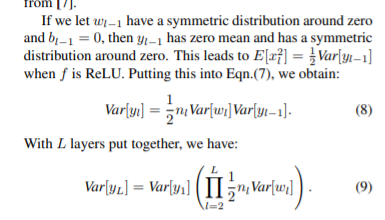# Kaiming in Lesson 8

In Lesson 8 Jeremy says the Kaiming init is to preserve mean,std=0,1 after applying lin+relu.
After getting mean 0.5 he tries to fix the relu to do (relu-0.5) to get a mean 0 after after applying the layer.
Im reading the 2.2 section of the paper and the formula that the init is derived from is (10):
0.5*nl*Var[wl] = 1 (for all l) so that (9) Var[yL] does not explode/vanish.
I cannot find in the paper where they want mean,std=0,1 after applying a lin+relu what am i missing?
If I understand correctly the paper doesnt state an objective of mean,std=0,1 so is this important and how?

a mean, std = 0,1 is a widely used (perhaps The most widely used) distribution in statistics. It has wonderful properties!

According to the BatchNorm paper, the authors suggest that normalization of layers reduces the “covariate shift” (some fancy mathematical concept, nevermind). But in lesson 6 , JH suggests that it doesn’t reduce the covariate shift, rather smoothens out the contours - makes them less bumpy, and hence result in faster, and smoother training, thus giving better results.

The same argument applies here. Its usually seen that if the initial batches are trained well, then the model is trained well(by that I mean, really well). So you’d want the layers to give a normalized output in the initial stages. That’s the rationale behind a specific kind of initialization of layers- you want them to give a normally distributed output.

In lesson 8, the distribution of the weights is not initialized as (mean, std)=(0,1). Rather the output of the layer+ReLU during forward propagation(or backward propagation, depending on how you’ve initiialized the layers) comes out to be of mean,std=0,1, when initialized by Kaiming method
Hope that helps

Thanks for the response.
“Rather the output of the layer+ReLU during forward propagation comes out to be of mean,std=0,1”
In the lesson we saw the mean of the output isnt 0 when initializing with kaiming and that bothered JH.
In the paper is seems the objective is to solve (10) and they dont aim to get mean 0 so I was wondering why is bothers JH.
Thanks!

@yonigo
The problem with a non-zero, and especially a larger non zero mean is that it leads to exploding values, ie, one layer pushes the mean to some positive/negative value, and the next pushes it further away. Finally the values are so large in magnitude, that the gradients too will be large in magnitude.This leads to divergence. I think that naturally too, training would push the weights such that the output of layers are closer to mean 0 ( something on the lines of regularization has, that is, balancing and controlling the weights - positives cancel negatives). When we initialize such that the output of layers are of mean 0, then we just facilitate learning.

Though I can’t cite any academic theory behind this intuition. Someone else might be able to give you a more concrete explanation.
But I hope it gives you a general idea behind this idea of initialization.

By the way, the authors of the paper DO derive the equation with the assumption that the weights are initialized with zero mean.